# SSC Quantitative Aptitude Questions (Day-73)

Dear Aspirants, you can find the Quantitative Aptitude questions with detailed explanations for the SSC exams. Nowadays the competitive level of the exam has been increasing consistently. Due to the great demand for the government job, the level of the toughness reached greater. Candidates have to enhance the preparation process in order to drive in the right path. It doesn’t need to clear the prescribed cutoff. You must have to score good marks more than the cut off marks to get into the final provisional list. Here we have updating the Quantitative Aptitude questions with detailed explanations on a daily basis. You can practice with us and measure your level of preparation. According to that you can sculpt yourself in a proper way. SSC aspirants kindly make use of it and grab your success in your career.

Start Quiz

1)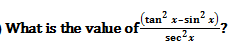(a) sin4 x

(b) cos2 x

(c) sin2 x

(d) cos4 x

2) If sin x =
1/3 and cos y = 1/3, then what is the value of sin (x + y)?

(a) 2/3

(b) 4/9

(c) 5/9

(d) 1

3)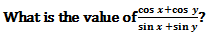(a) tan ((x+y)/2)

(b) tan ((x-y)/2)

(c) cot ((x-y)/2)

(d) cot ((x + y)/2)

4) What is the value of sec12° sin12° tan38° tan78° tan52°?

(a) 1

(b) 3

(c) 1/2

(d) 3/2

5)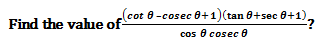(a) 2 cos θ

(b) 2

(c) 2 cot θ

(d) 2 tan θ

Direction (6-10): The given pie chart shows the various expenses (in percent) incurred by publisher on publishing a book.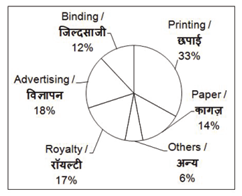6) If the total amount spend on publishing the book is Rs 68000, then what will be the amount (in Rs) spend on Advertising?

(a) 11560

(b) 12240

(c) 9520

(d) 8160

7) If the amount spend on Binding is Rs 14400, then what will be the amount (in Rs) spend on Paper?

(a) 7200

(b) 20400

(c) 15600

(d) 16800

8) By how much percent the total amount spend on Paper and Binding is less than the amount spend on Printing?

(a) 21.21

(b) 45.45

(c) 30.3

(d) 33.33

9) By how much the average amount spend on Printing, Paper and Royalty is more (in Rs) than the average amount spend on Advertising and Binding if total amountspend on Publication is Rs 150000?

(a) 9500

(b) 10000

(c) 7500

(d) 9000

10) For 11000 books the expenses incurred on others is Rs 36960. If publisher wants a profit of 25%, then what should be the marked price (in Rs) of each book?

(a) 56

(b) 76

(c) 70

(d) 50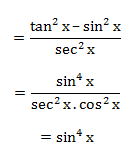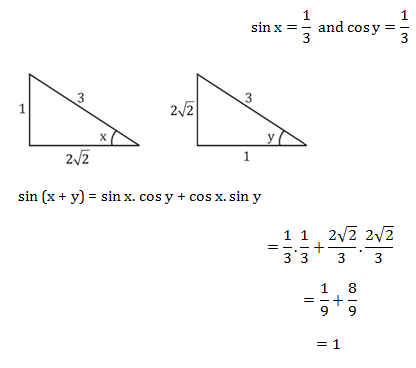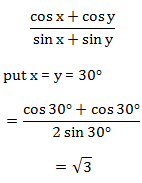Only option (d) satisfies the given equation.

Tan 12°. Tan 38°. Tan 78°. Tan 52°.

= Cot 78°. Cot 52°. Tan 78°. Tan 52°

= 1

Put θ = 30°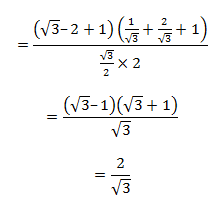Only option (d) satisfies the given expression.

= 18/100 × 68000

= 12240

If 12% of given = 14400

Then amount spend on paper will be

= 14400/12 × 14

= 16800

Paper & binding = 26%

Amount spend on Printing = 33%

= (33 –26)/33 × 100%

= 7/33 × 100% = 21.21%

Printing, paper & Royalty

= 33 + 14 + 17

= 64%

Avg. = 64/3%

Amount on Advertising & Binding = 30%

Avg.= 30/2=15%

Difference = (21.33 – 15)%

= 19/3%

Amount = 150000× 19/300

= 9 500

Others 6% = 36960

Total = 616000

M.P. of each book = 56 × 5/4

= 14 × 5

= 70Rs.

 Check Here to View SSC CGL / CHSL 2021 Quantitative Aptitude Questions Day – 72 Day – 71 Day – 70